# 8.1 through 8.3 Draw the influence lines for vertical reactions at supports A and C and the shear and bending moment at point B of the beams shown in Figs. P8.1 through P8.3. FIG.P8.1, P8.59BuyFind

### Structural Analysis

6th Edition
KASSIMALI + 1 other
Publisher: Cengage,
ISBN: 9781337630931BuyFind

### Structural Analysis

6th Edition
KASSIMALI + 1 other
Publisher: Cengage,
ISBN: 9781337630931

#### Solutions

Chapter 8, Problem 1P
Textbook Problem

## 8.1 through 8.3 Draw the influence lines for vertical reactions at supports A and C and the shear and bending moment at point B of the beams shown in Figs. P8.1 through P8.3.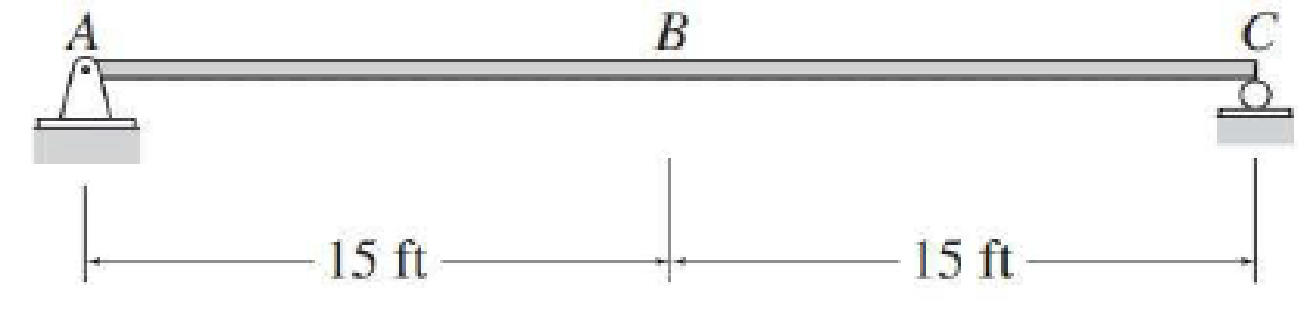FIG.P8.1, P8.59

Expert Solution
To determine

Draw the influence lines for the vertical reactions at supports A and C.

Draw the influence lines for the shear and bending moment at point B.

### Explanation of Solution

Calculation:

Apply a 1 k unit moving load at a distance of x from left end A.

Sketch the free body diagram of beam as shown in Figure 1.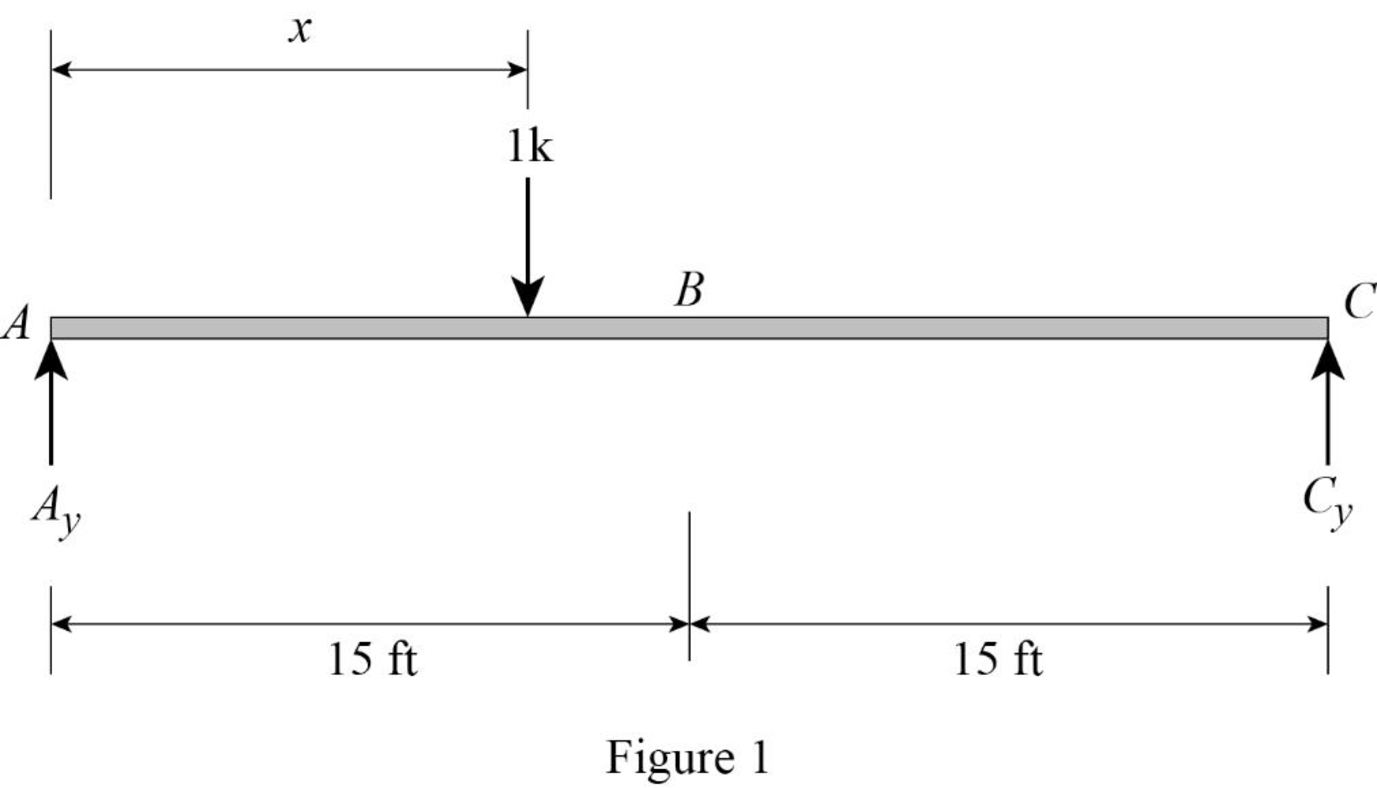Refer Figure 1.

Find the equation of support reaction (Ay) at A using equilibrium equation:

Take moment about point C.

Consider moment equilibrium at point C.

Consider clockwise moment as positive and anticlockwise moment as negative

Sum of moment at point C is zero.

ΣMC=0Ay(30)1(30x)=0Ay(30)30+x=030Ay=30xAy=1x30 (1)

Find the equation of support reaction (Cy) at C using equilibrium equation:

Apply vertical equilibrium equation of forces.

Consider upward force as positive (+) and downward force as negative ().

Ay+Cy=1

Substitute 1x30 for Ay.

1x30+Cy=1Cy=11+x30Cy=x30 (2)

Consider Equation (1).

Find the value of influence line ordinate of reaction Ay at support A.

Substitute 0 for x in Equation (1).

Ay=1030=1k/k

Similarly calculate the influence line ordinate of reaction Ay for different value of x and summarize the result in Table 1.

 x (ft) Ay (k/k) 0 1 15 0.5 30 0

Draw the influence line diagram for the vertical reactions at support A using Table 1 as shown in Figure 2.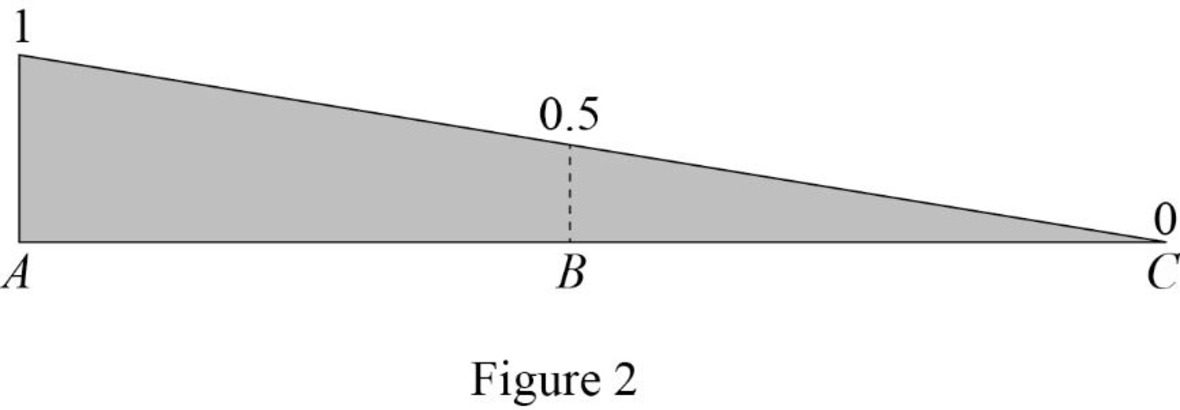Consider Equation (2).

Find the influence line ordinate of reaction Cy at support C.

Substitute 30 for x in Equation (2).

Cy=3030=1

Similarly calculate the influence line ordinate of reaction Cy for different value of x and summarize the result in Table 2.

 x (ft) Cy (k/k) 0 0 15 0.5 30 1

Draw the influence line diagram for the vertical reactions at support C using Table 2 as shown in Figure 3.Find the equation of shear force at B of portion AB (0x<15ft).

Sketch the free body diagram of the section AB as shown in Figure 4.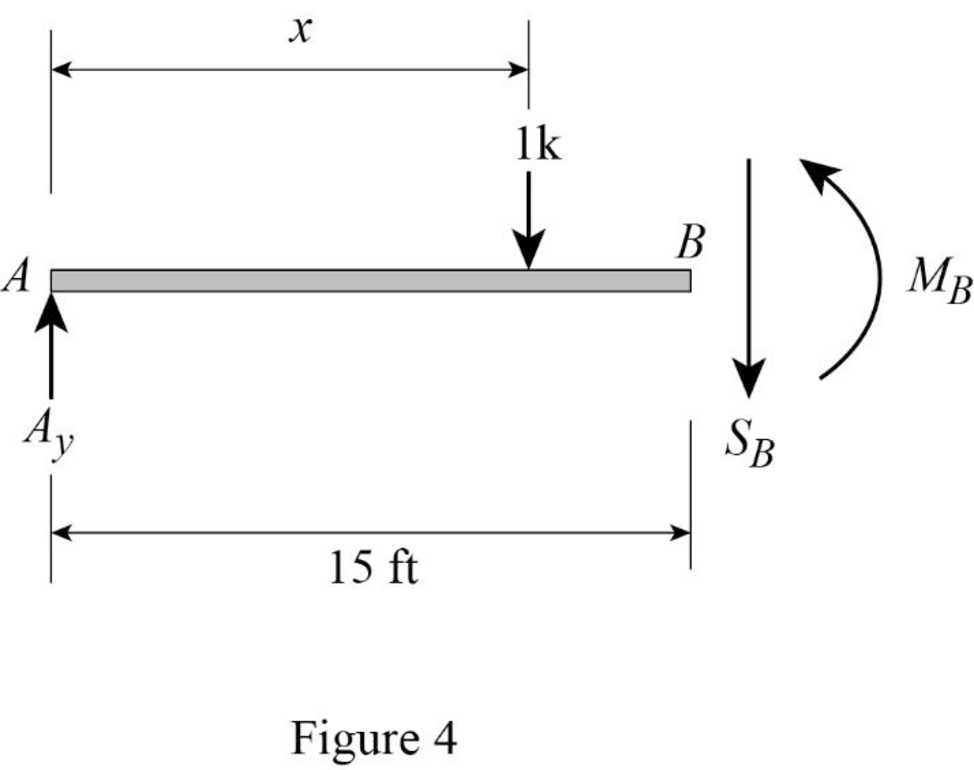Refer Figure 4.

Apply equilibrium equation of forces.

Consider upward force as positive (+) and downward force as negative ().

ΣFy=0

AySB1=0SB=Ay1

Substitute 1x30 for Ay.

SB=1x301=x30

Find the equation of shear force at B of portion BC (15ft<x30ft).

Sketch the free body diagram of the section BC as shown in Figure 5.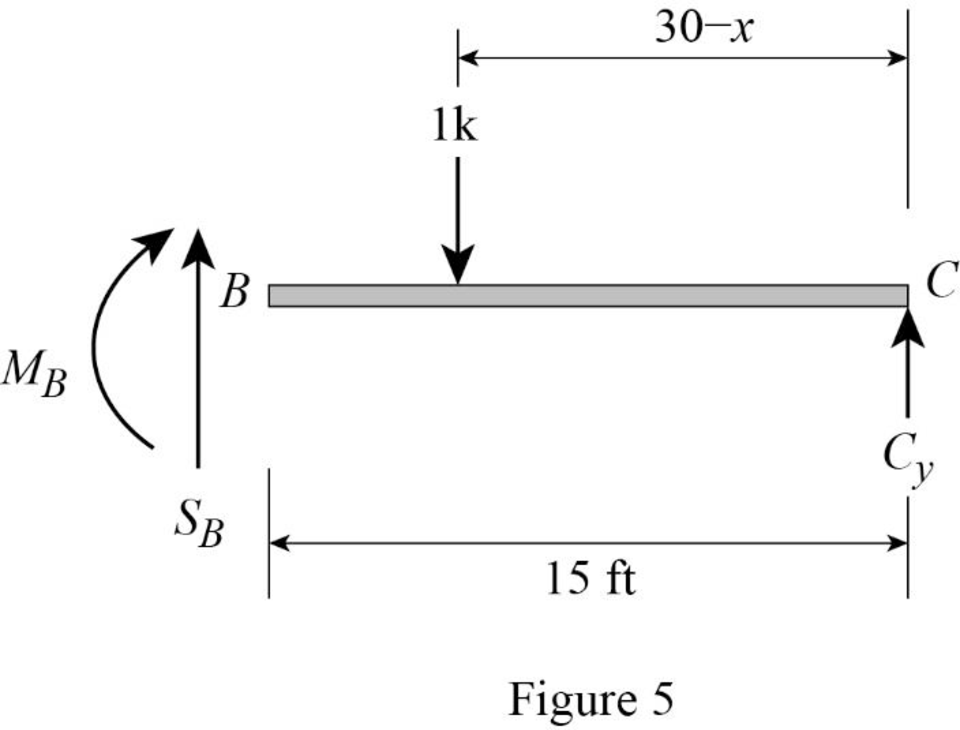Refer Figure 5.

Apply equilibrium equation of forces.

Consider upward force as positive (+) and downward force as negative ().

ΣFy=0

SB1+Cy=0SB=1Cy

Substitute x30 for Cy.

SB=1x30

Thus, the equations of the influence line for SB are,

SB=x30 0x<15ft (3)

SB=1x30 15ft<x30ft (4)

Find the value of influence line ordinate of shear force at various points of x using the Equations (3) and (4) and summarize the value as in Table 3.

 x (ft) SB (k/k) 0 1 15− −12 15+ 12 30 0

Draw the influence lines for the shear force at point B using Table 3 as shown in Figure 6.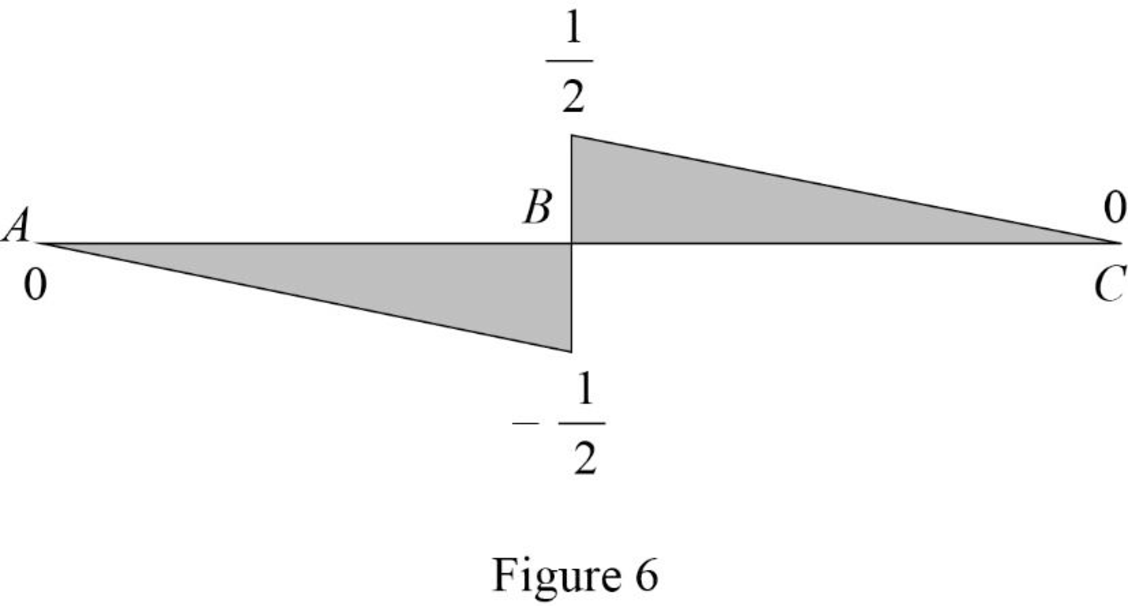Refer Figure 4.

Consider clockwise moment as positive and anticlockwise moment as negative.

Find the equation of moment at B of portion AB (0x<15ft).

MB=Ay(15)(1)(15x)

Substitute 1x30 for Ay.

MB=(1x30)(15)(1)(15x)=15x215+x=x2

Refer Figure 5.

Consider clockwise moment as negative and anticlockwise moment as positive.

Find the equation of moment at B of portion BC (15ft<x30ft).

MB=Cy(15)(1)[15(30x)]=15Cy15+(30x)=15Cy+15x

Substitute x30 for Cy.

MB=15(x30)+15x=x2+15x=15x2

Thus, the equations of the influence line for MB are,

MB=x2 0x<15ft (5)

MB=15x2 15ft<x30ft (6)

Find the value of influence line ordinate of moment at various points of x using the Equations (5) and (6) and summarize the value as in Table 4.

 x (ft) MB (k-ft/k) 0 0 15 7.5 30 0

Draw the influence lines for the moment at point B using Table 4 as shown in Figure 7.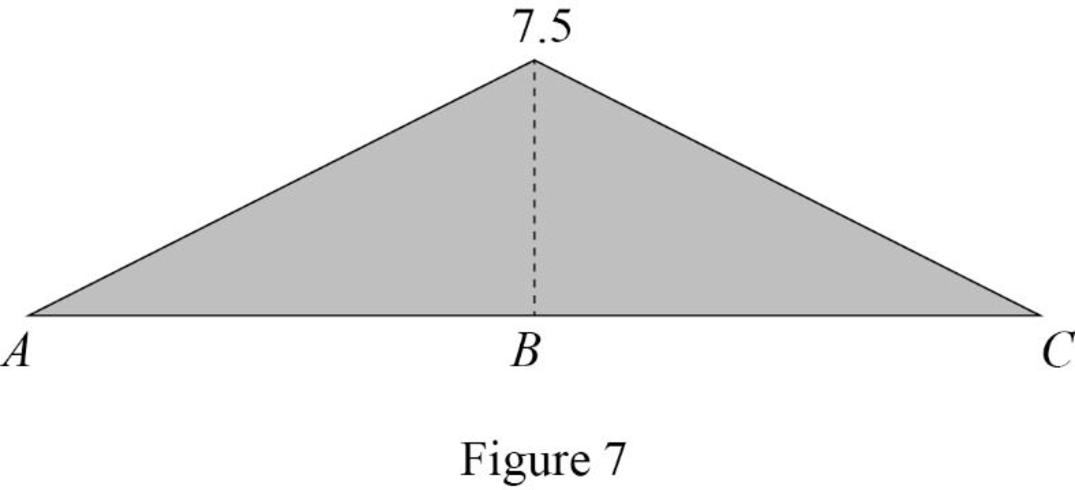Therefore, the influence lines for the vertical reactions at supports A and C and the influence lines for the shear and bending moment at point B are drawn.

### Want to see more full solutions like this?

Subscribe now to access step-by-step solutions to millions of textbook problems written by subject matter experts!

### Want to see more full solutions like this?

Subscribe now to access step-by-step solutions to millions of textbook problems written by subject matter experts!© 2021 bartleby. All Rights Reserved.